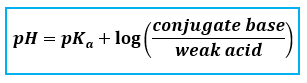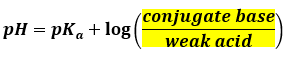# Problem: You need to prepare 100.0 mL of a pH = 4.00 buffer solution using 0.100 M benzoic acid (pKa = 4.20) and 0.180 M sodium benzoate. How much of each solution should be mixed to prepare this buffer?

###### FREE Expert Solution

Since we are dealing with a buffer solution, we are going to use the Henderson-Hasselbalch equation:We need to know the volume of the acid (VA) and the conjugate base (VBrequired to prepare the buffer. But first we have to calculate the ratio of the conjugate base and the weak acid:

benzoic acid is a weak acid

benzoate in sodium benzoate is the conjugate base

- concentration of benzoate = concentration of sodium benzoate###### Problem Details

You need to prepare 100.0 mL of a pH = 4.00 buffer solution using 0.100 M benzoic acid (pKa = 4.20) and 0.180 M sodium benzoate. How much of each solution should be mixed to prepare this buffer?